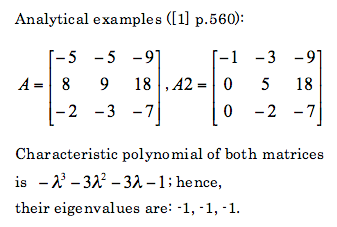## Wednesday, January 09, 2008

### Python: eigenvalues with Scipy and Sympy

Eigenvalues and eigenvectors are important in systems of differential equations. To speed up my analysis I decided to use some numerical package for getting eigenvalues form square matrices. I found two numerical packages Scipy and Sympy for Python. Both have ability to calculate eigenvalues. But their calculations are not reliable. I present few very simple examples showing how rubbish these packages are.Lets see what does Python calculate:
Scipy
`from scipy import *from scipy.linalg import *A=matrix([ [-5,-5,-9],[8,9,18],[-2,-3,-7] ])print eigvals(A)# [-0.999989 +1.90461984e-05j -0.999989 -1.90461984e-05j# -1.00002199 +0.00000000e+00j]A2=matrix([ [-5,-5,-9],[8,9,18],[-2,-3,-7] ])print eigvals(A2)# [-1.+0.j 　　-1.00000005+0.j 　　-0.99999995+0.j]`
Sympy
`from sympy import *from sympy.matrices import Matrixx=Symbol('x')A=Matrix(( [-5,-5,-9],[8,9,18],[-2,-3,-7] ))print roots(A.charpoly(x),x)# Returns error!!!A2=Matrix(( [-1,-3,-9],[0,5,18],[0,-2,-7] ))print roots(A2.charpoly(x),x)# Returns error!!!`
Based on these to examples it is clearly seen that these two packaged are unreliable, at least as far as calculation of eigenvalues is considered.

For matrices 2x2 it was noticed that the eigenvalues are correctly calculated both for real and complex values.

For comparison lets check what does Matlab returns:`A=[-5,-5,-9;8,9,18;-2,-3,-7]eig(A)ans =-1.0000 + 0.0000i-1.0000 - 0.0000i-1.0000A2=[-5,-5,-9;8,9,18;-2,-3,-7]eig(A2)ans =-1.0000 + 0.0000i-1.0000 - 0.0000i-1.0000`
So it is seen that Matlab does correctly calculate these eigenvalues.

Even if one manually calculates characteristic polynomial and would like to gets roots of it, I would recommend neither Scipy or Sympy. As an example I calculate roots of the previously obtained characteristic polynomial for matrices A and A2:
`import scipy as scp=sc.poly1d([-1,-3,-3,-1])print p= sc.roots(p)# [-1.0000086 +0.00000000e+00j -0.9999957 +7.44736442e-06j# -0.9999957 -7.44736442e-06j]``import sympy as syx=sy.Symbol('x')print sy.solve(-x**3-3*x**2-3*x-1==0,x)#[-1]`
As can be seen calculation of roots of polynomials is also not good. In the second case there is one root (-1) instead of three (-1,-1,-1).

For comparison lets check Matlab (or Octave 3):`p=[-1,-3,-3,-1]roots(p)ans =-1.0000 -1.0000 + 0.0000i-1.0000 - 0.0000i`

Based on these results it is seen that its better to use Matlab for calculation of eignevalues.

References:
 S.I. Grossman, Multivariable calculus, linear algebra and differential equation, Second edition, 1986

1.2.from sympy import *
from sympy.matrices import Matrix
x=Symbol('x')
A=Matrix(( [-5,-5,-9],[8,9,18],[-2,-3,-7] ))
A=A - x*A*A.inv()
g=det(A)
roots(g,x)

3.me equivoque...se escribia A.det() XD

4.Sorry but I do not understand your conclusions. From the results you show, it is clear that Matlab limits its output to the fourth digit after the comma (1.000), while Python prints more digits. If in the first example (SciPy) you limit the printout to 4 digits as Matlab:

["%.4f" % x for x in eigvals(A)]

you will get
ans = ['-1.0000', '-1.0000', '-1.0000']

which is exactly the same output as Matlab.

By the way, I ran Gnu R and a C program written using the GSL, and they all returned more or less the same values as SciPy (i.e. eigenvalues which differ from 1.0 for roughly 1e-5 and have an imaginary part which is of the same magnitude). So to me there is no clear evidence that SciPy is so bad.

1.Anonymous3:42 AM

Indeed, in Matlab:

>> format long
>> A=[-5,-5,-9;8,9,18;-2,-3,-7];
>> eig(A)

ans =

-0.999990315867095 + 0.000016774094561i
-0.999990315867095 - 0.000016774094561i
-1.000019368265816

>> A2=[-1,-3,-9;0,5,18;0,-2,-7];
>> eig(A2)

ans =

-1.000000000000000
-1.000000064523922
-0.999999935476080

5.Anonymous7:10 AM

For sympy version 0.6.7 I get:
>>> A=Matrix([ [-5,-5,-9],[8,9,18],[-2,-3,-7] ])
>>> p = A.charpoly(x)
>>> print roots(p,x)
{-1: 3}

i.e. three times -1.